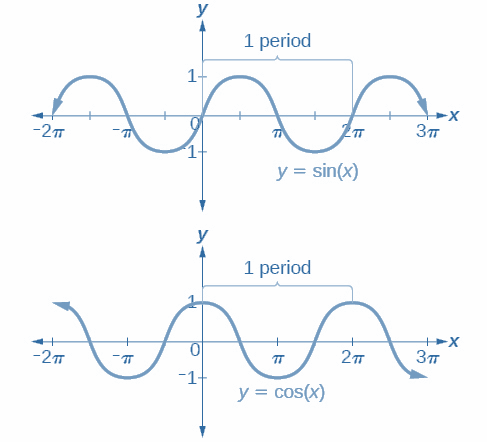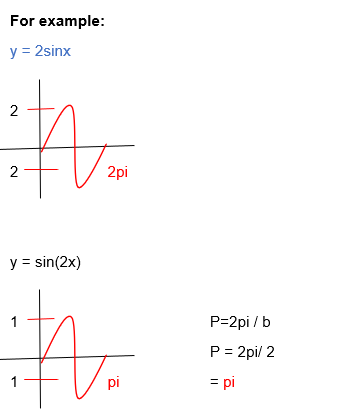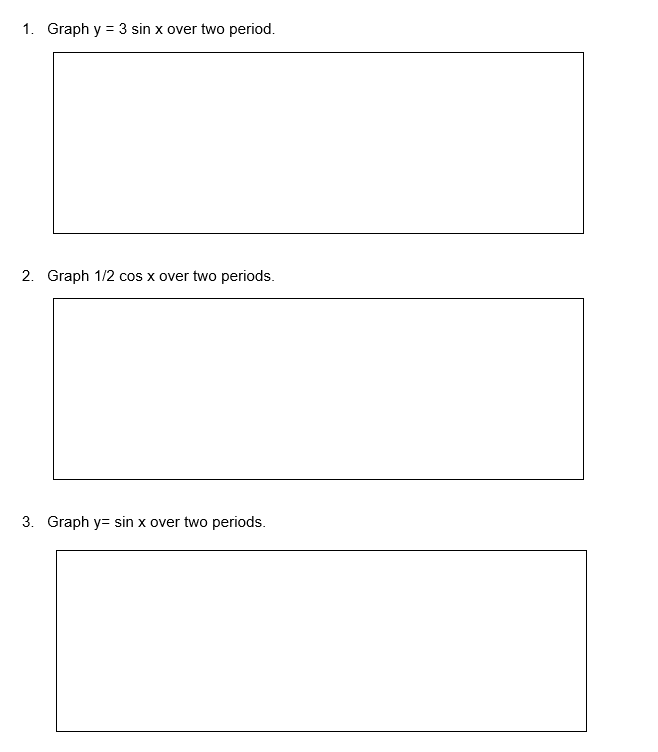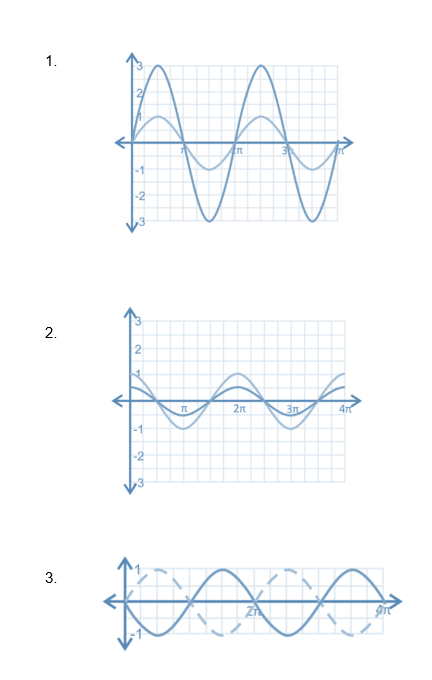# Graphing Sine and Cosine Worksheet (with answer key +PDF)

The horizontal transformations of the sine and cosine graphs. Using the cofunction identity and the cosine negative angle identity, we can demonstrate this. Negative Angle Identity: sin(θ) = cos (π2−θ) Cofunction Identity: cos(−θ+π2) =cos (−(θ−π2)) =cos(θ−π2).

## What is the “Graphing Sine and Cosine Worksheet (with answer key +PDF)”?

This worksheet will explore some of the Graphing sine and cosine, which is the information provided in this worksheet.

## What are Graph sine and cosine?

The unit circle will be translated from the trigonometric ratio to the angle in radians in the Cartesian coordinate system in order to graph the sine and cosine functions. The unit circle will be “unraveled” in order to complete this process.

This worksheet will help you solve and graph sine and cosines. In this lesson, the students are enabled to answer the following exercises below.

Instructions on how to use the “Graphing Sine and Cosine Worksheet (with answer key +PDF).”

Study the concept and examples given and try to solve the exercises below.

## Conclusion

The sine and cosine graphs both have the same shape, which is a recurring “hill and valley” pattern over a length-based interval on the horizontal axis. The real numbers serve as both the sine and cosine functions’ domain and range, respectively.

If you have any inquiries or feedback, please let us know.

## Graphing Sine and Cosine Worksheet (with answer key +PDF)

Graphing Sine and Cosine## Worksheet

Show the graphs of sine and cosine.